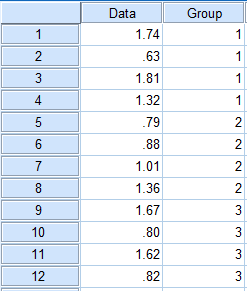Your browser doesn't support the features required by impress.js, so you are presented with a simplified version of this presentation.

For the best experience please use the latest Chrome, Safari or Firefox browser.

# From 2-sample t Test to ANOVA

• 2-sample t test compares the means of two populations
• ANOVA generalizes this to more than two populations
• $H_0:\mu_1=\mu_2=\cdots=\mu_I$, i.e., all the population means are the same
• $H_a:$ not all of the $\mu_i$ are equal
• $H_a$ is NOT $\mu_1\neq\mu_2\neq\cdots\neq\mu_I$

# The Pooled Variance

• We usually assume that the (population) variances for each group are the same. To check this, use the following rule:
• If the largest std. is less than twice the smallest standard deviation, we can claim that the assumption is valid.

# $R^2$ and $s$

• $R^2=$ Sum of Squares Between Groups / Total Sum of Squares
• $s=\sqrt{\text{Mean Square Within Groups}}$

# Multiple Comparisons

• If $H_0$ is rejected, we only know that some of the means are different.
• To find out the pairs that have significant differences, we need to do the multiple comparisons using Bonferroni method.
• P-value less than $\alpha$ means that the corresponding pair has significant difference.

# In SPSS

• Data format for ANOVA is similar to 2-sample t test: one column for data, one column for group.• Input number code for each fish category, e.g. 1 for largemouth bass, 2 for catfish, 3 for trout, 4 for walleye.